Next: Using KL as a Up: Karhunen-Loeve Decomposition for Statistical Previous: Decoding a Key into

## Varying n for Speed or Resolution

Note that the original vectors and the eigenvectors are all n-tuples with n=7000. Therefore, the computation of the 60-dimensional key (c0, c1, ..., c59) given by Equationrequires convolution of the input image (an arbitrary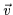n-tuple) with 60 eigenvectors (the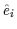n-tuples). Thus, 60 convolutions of 7000 pixel masks must be performed each time to convert an image into its key. To reduce this computation, we have also generated scaled versions of the input vectors and eigenvectors. These images are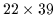pixels and require n=858 element vectors. However, the computation of (c0, c1,..., c59) for the smaller vectors requires roughly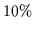of the original processing time. The mean face for the smaller data set is shown in Figure. Thus, these versions of the KL decomposition can be useful when time is more critical than resolution or precision. However, the quality of such low resolution images makes them unreliable for face recognition purposes. These should only be used to perform coarse face detection. We shall now describe a technique for detecting a face using an image's 60-scalar KL code.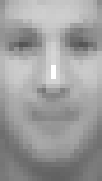Next: Using KL as a Up: Karhunen-Loeve Decomposition for Statistical Previous: Decoding a Key into
Tony Jebara
2000-06-23